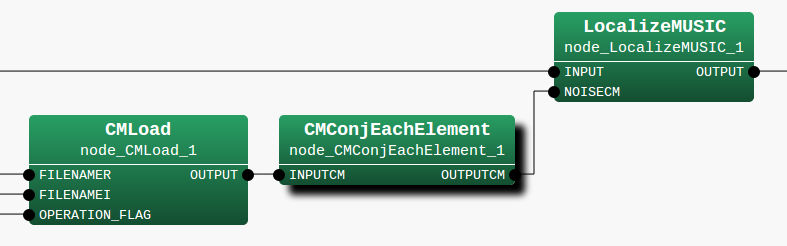## 6.2.8 CMConjEachElement

### 6.2.8.1 Module Overview

Performs conjugate of a correlation matrix.

None.

### 6.2.8.3 Usage

In what case is the node used?

The calculation node of the correlation matrix is the one created for the sound source from CMMakerFromFFT , CMMakerFromFFTwithFlag , and has the function of taking conjugate of each component.

Typical Examples

Figure. 6.24 shows the usage example of CMConjEachElement  node. The input terminal is connected to a correlation matrix calculated from CMMakerFromFFT  or CMMakerFromFFTwithFlag , etc. (type is Matrix<complex<float> >  type, but to handle a correlation matrix, convert the three dimensional complex array to a two dimensional complex array and then output).Figure 6.24: Network Example using CMConjEachElement

### 6.2.8.4 I/O and property setting of the node

Input

INPUTCM

: Matrix<complex<float> >  type. A correlation matrix for each frequency bin. The $M$-th order complex square array correlation matrix inputs $NFFT/2 + 1$ items. Matrix<complex<float> >  contains rows corresponding to frequency ($NFFT/2 + 1$ rows), and columns containing the complex correlation matrix ($M * M$ columns across).

Output

OUTPUTCM

: Matrix<complex<float> >  type. The correlation matrix after taking conjugate of INPUTCM is output.

Parameter

No parameters.

### 6.2.8.5 Module Description

Performs conjugate of a correlation matrix. The correlation matrix is a complex three-dimensional array of size $k \times M \times M$ and the division $k \times M \times M$ times is performed as follows. Here, $k$ is the number of frequency bins ($k = NFFT/2 + 1$), and $M$ is the number of channels in the input signal.


calculate{

FOR i = 1 to k
FOR i = 1 to M
FOR i = 1 to M

OUTPUTCM[i][j][k] = conj(INPUTCM[i][j][k])

ENDFOR
ENDFOR
ENDFOR

}